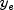JMSLTM Numerical Library 7.2.0
com.imsl.math

## Interface NumericalDerivatives.Function

• ### Method Summary

Methods
Modifier and Type Method and Description
`double[]` ```f(int varIndex, double[] y)```
Returns the equations evaluated at the point `y`.
• ### Method Detail

• #### f

```double[] f(int varIndex,
double[] y)```
Returns the equations evaluated at the point `y`. If the user does not override the `evaluateF` method, then `f` is also used to compute the function values at the current independent variable values.
Parameters:
`varIndex` - an `int` indicating the index of the variable to perturb. `varIndex` = 1 indicates variable 1 in `y`.
`y` - a `double` array of length n, the point at which the Jacobian is to be evaluated.
Returns:
a `double` array of length m. The equations evaluated at the point `y`.
JMSLTM Numerical Library 7.2.0